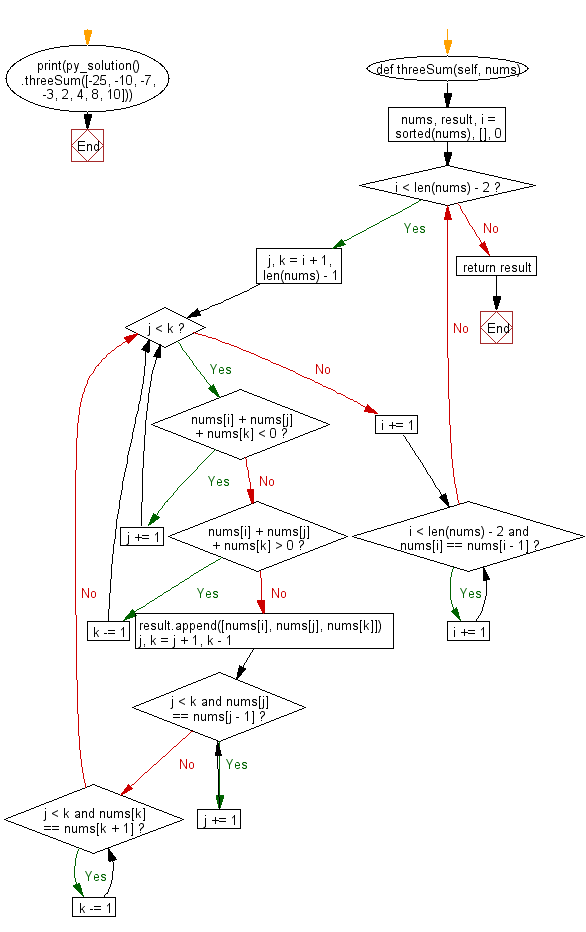﻿ Python: Find the three elements that sum to zero from a set of n real numbers - w3resource# Python: Find the three elements that sum to zero from a set of n real numbers

## Python Class: Exercise-6 with Solution

Write a Python class to find the three elements that sum to zero from a set (array) of n real numbers.

Sample Solution:

Python Code:

``````class py_solution:
def threeSum(self, nums):
nums, result, i = sorted(nums), [], 0
while i < len(nums) - 2:
j, k = i + 1, len(nums) - 1
while j < k:
if nums[i] + nums[j] + nums[k] < 0:
j += 1
elif nums[i] + nums[j] + nums[k] > 0:
k -= 1
else:
result.append([nums[i], nums[j], nums[k]])
j, k = j + 1, k - 1
while j < k and nums[j] == nums[j - 1]:
j += 1
while j < k and nums[k] == nums[k + 1]:
k -= 1
i += 1
while i < len(nums) - 2 and nums[i] == nums[i - 1]:
i += 1
return result

print(py_solution().threeSum([-25, -10, -7, -3, 2, 4, 8, 10]))
```
```

Sample Output:

```[[-10, 2, 8], [-7, -3, 10]]
```

Pictorial Presentation:Flowchart:## Visualize Python code execution:

The following tool visualize what the computer is doing step-by-step as it executes the said program:

Python Code Editor:

What is the difficulty level of this exercise?

Test your Programming skills with w3resource's quiz.

﻿

## Python: Tips of the Day

The Zip() Function:

```>>> students = ('John', 'Mary', 'Mike')
>>> ages = (15, 17, 16)
>>> scores = (90, 88, 82, 17, 14)
>>> for student, age, score in zip(students, ages, scores):
...     print(f'{student}, age: {age}, score: {score}')
...
John, age: 15, score: 90
Mary, age: 17, score: 88
Mike, age: 16, score: 82
>>> zipped = zip(students, ages, scores)
>>> a, b, c = zip(*zipped)
>>> print(b)
(15, 17, 16)
```